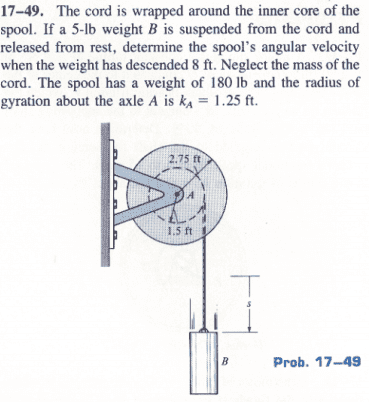## Homework Statement## The Attempt at a Solution

Alright, so the solution manual shows that the intertia of the disk is:
I = m * k2, where k is the radius of gyration​

However why can't the inertia of the disk be:
I = 0.5 * m * r2, where r is the radius of the disk​
This is the formula for the inertia of a rotating disk... However when I plug in all my numbers I get a different inertia value when compared to the inertia value that I got using the radius of gyration.

haruspex